## 4.2 Ionic and total abundances from ORLs

Using the effective recombination coefficients (given in Table 4), we determine ionic abundances, X/H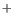, from the measured intensities of optical recombination lines (ORLs) as follows:(1)

where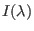is the intrinsic line flux of the emission line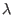emitted by ion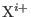,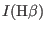is the intrinsic line flux of H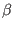,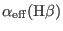the effective recombination coefficient of H, and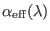the effective recombination coefficient for the emission line.

Abundances of helium and carbon from ORLs are given in Table 6. We derived the ionic and total helium abundances from HeI4471,5876 and6678 lines. We assumed the Case B recombination for the HeI lines (Porter et al., 2012; Porter et al., 2013). We adopted an electron temperature of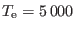K from HeI lines, and an electron density of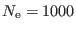cm. We averaged the He/Hionic abundances from the HeI4471,5876 and6678 lines with weights of 1:3:1, roughly the intrinsic intensity ratios of these three lines. The total He/H abundance ratio is obtained by simply taking the sum of He/Hand He/H. However, He/His equal to zero, since HeII4686 is not present. The C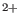ionic abundance is obtained from C II6462 and7236 lines.

 Ion(Å) Mult ValueHe4471.50 V14 0.141 5876.66 V11 0.121 6678.16 V46 0.115 Mean 0.124 He4685.68 3.4 0.0 He/H 0.124 C6461.95 V17.40 3.068(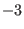) 7236.42 V3 1.254() Mean 2.161()AssumingK and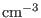.

Ashkbiz Danehkar
2018-03-26